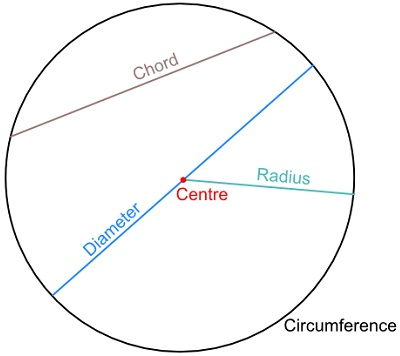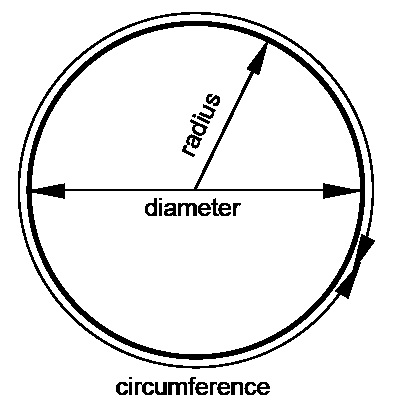# Circle Area Calculator

Circle area calculator tool is used to calculate circumference, diameter, area of the circle, if the radius is given. Here, in the tool enter the radius of the circle to calculate all parameters.

In a circle, all the points are equidistant from the given point called the centre of the circle. The distance from the centre of the circle to the longest chord (diameter) is zero.## FormulasThe formula for the area of a circle is: π r2

The formula for the circumference of a circle is: 2 x π x r

The formula for the diameter of a circle is : 2 x r

### What is a Circle?

A round plane figure whose boundary (the circumference) consists of points equidistant from a fixed point (the centre).

#### Center of a Circle

A point in the circle and is at equal distance from all points on the circumference.

A line segment with one endpoint on the center and the other end point on the circle or circumference.

#### Diameter

A line segment that passes through the center of a circle and has endpoints on the circle.

#### π (Pi)

In math, there are numbers that come up so often, we give them their own symbol. One such special number is π(pi).

The symbol for pi is π. And the value of π is 3.14 (approx).

The number shows up as the ratio of a circle’s circumference to its diameter. The circumference is the distance around the circle and the diameter is the distance across the circle through the center. Another dimension we will often mention is the radius of a circle. The radius is half the length of the diameter. This means the diameter is twice the length of the radius.